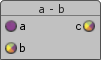## FANDOM

167 Pages## NameEdit

a - b - returns vector c, which is a result of subtracting vector a & vector b

## SynopsisEdit

```vector1 subtract(vector1 a, vector1 b);
vector2 subtract(vector1 a, vector2 b);
vector3 subtract(vector1 a, vector3 b);
vector4 subtract(vector1 a, vector4 b);
```

## ParametersEdit

a
A value.
b
A value.
c
The returning value of subtraction.

## DescriptionEdit

Returns c = a - b.

For vectors, the returned vector c contains difference of each element of the vector (only vector1) and the respective element of the vector b (vector1-vector4).